### Pengiun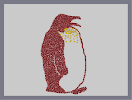Hover over the thumbnail for a full-size version.

Author theninjagame author:theninjagame n-art rated 2005-12-20 4 by 20 people. \$Pengiun#theninjagame#none#00000000000000000000000000000000000000000000000000000000000000000000000000000000000000000000000000000000000000000000000000000000000000000000000000000000000000000000000000000000000000000000000000000000000000000000000000000000000000000000000000000000000000000000000000000000000000000000000000000000000000000000000000000000000000000000000000000000000000000000000000000000000000000000000000000000000000000000000000000000000000000000000000000000000000000000000000000000000000000000000000000000000000000000000000000000000000000000000000000000000000000000000000000000000000000000000000000000000000000000000000000000000000000000000000000000000000000000000000000000000000000000000000000000000000000000000000000000000000000|12^483,158!12^478,153!12^476,156!12^471,151!12^461,147!12^467,152!12^458,153!12^455,150!12^454,150!12^452,148!12^449,148!12^434,148!12^432,151!12^448,151!12^443,148!12^436,144!12^446,143!12^456,145!12^454,141!12^436,141!12^429,145!12^424,149!12^422,144!12^445,113!12^437,115!12^426,119!12^425,122!12^423,126!12^420,132!12^418,135!12^416,138!12^424,104!12^434,102!12^439,100!12^445,100!12^451,100!12^460,100!12^467,100!12^475,101!12^477,103!12^478,106!12^479,107!12^482,111!12^482,113!12^469,109!12^462,109!12^454,111!12^475,111!12^477,111!12^469,103!12^463,103!12^462,107!12^449,106!12^444,105!12^440,110!12^454,107!12^454,104!12^440,105!12^431,107!12^430,116!12^430,121!12^434,109!12^427,113!12^423,118!12^421,118!12^423,149!12^419,153!12^418,157!12^422,157!12^427,160!12^413,147!12^423,110!12^421,110!12^416,121!12^414,127!12^406,132!12^406,140!12^406,148!12^413,159!12^413,151!12^414,154!12^409,138!12^412,134!12^412,146!12^412,142!12^413,141!12^431,99!12^422,102!12^420,101!12^417,101!12^420,97!12^419,97!12^413,97!12^408,97!12^404,94!12^402,94!12^397,92!12^393,92!12^384,91!12^379,87!12^377,87!12^374,87!12^369,87!12^358,88!12^353,90!12^336,108!12^332,113!12^329,118!12^326,121!12^323,126!12^320,132!12^317,138!12^316,144!12^312,150!12^310,156!12^310,158!12^350,95!12^339,101!12^363,86!12^345,99!12^350,94!12^392,91!12^386,91!12^385,88!12^316,162!12^324,162!12^339,166!12^346,169!12^365,171!12^372,170!12^385,170!12^389,170!12^395,168!12^407,167!12^411,164!12^411,164!12^395,166!12^381,168!12^365,168!12^348,168!12^378,173!12^379,177!12^383,180!12^385,186!12^381,191!12^376,191!12^371,191!12^366,189!12^361,188!12^356,185!12^355,185!12^350,185!12^343,188!12^348,195!12^361,170!12^356,170!12^348,170!12^341,165!12^334,165!12^331,163!12^416,143!12^443,148!12^440,143!12^444,143!12^449,141!12^443,141!12^439,139!12^434,139!12^428,139!12^425,139!12^420,139!12^462,151!12^471,149!12^462,143!12^460,143!12^420,148!12^420,148!12^410,145!12^409,143!12^423,129!12^419,128!12^418,129!12^420,123!12^424,113!12^418,104!12^418,104!12^418,117!12^414,123!12^408,127!12^406,129!12^418,114!12^415,107!12^411,111!12^408,121!12^409,116!12^409,106!12^406,101!12^406,100!12^406,100!12^405,116!12^404,123!12^404,135!12^404,151!12^411,110!12^412,117!12^414,117!12^410,101!12^406,102!12^403,101!12^399,99!12^395,99!12^390,98!12^388,98!12^383,99!12^379,98!12^377,98!12^391,111!12^394,111!12^411,111!12^406,108!12^405,108!12^395,108!12^393,106!12^397,105!12^402,113!12^401,116!12^399,122!12^399,109!12^399,106!12^405,112!12^419,112!12^426,107!12^423,99!12^425,99!12^407,156!12^406,157!12^400,157!12^387,161!12^386,161!12^409,160!12^403,162!12^402,163!12^407,168!12^404,163!12^397,163!12^394,161!12^400,156!12^406,151!12^403,146!12^396,154!12^395,147!12^400,139!12^399,139!12^404,126!12^397,127!12^394,133!12^394,131!12^401,124!12^400,139!12^400,141!12^400,123!12^396,128!12^397,135!12^396,128!12^393,118!12^395,125!12^392,113!12^388,108!12^390,106!12^382,114!12^382,114!12^382,111!12^382,105!12^382,104!12^379,101!12^377,94!12^377,95!12^376,95!12^395,137!12^405,135!12^401,130!12^398,130!12^397,120!12^393,113!12^385,116!12^384,123!12^384,129!12^386,136!12^386,141!12^388,143!12^395,146!12^394,140!12^392,134!12^389,125!12^388,117!12^388,113!12^388,106!12^381,95!12^374,95!12^373,95!12^363,95!12^360,97!12^357,100!12^351,104!12^345,113!12^340,119!12^333,132!12^326,140!12^323,147!12^322,151!12^321,152!12^317,153!12^315,153!12^317,141!12^319,136!12^323,132!12^325,130!12^327,127!12^332,122!12^337,119!12^338,117!12^342,111!12^346,107!12^346,106!12^356,96!12^356,95!12^360,91!12^361,91!12^347,98!12^339,102!12^335,107!12^333,120!12^326,135!12^321,148!12^320,155!12^328,155!12^338,155!12^342,155!12^349,156!12^363,163!12^366,165!12^375,165!12^385,164!12^385,161!12^388,161!12^391,161!12^390,155!12^390,154!12^388,150!12^386,146!12^384,143!12^384,138!12^381,132!12^381,126!12^380,116!12^379,110!12^376,105!12^373,101!12^369,97!12^367,97!12^367,98!12^367,104!12^367,110!12^368,115!12^369,118!12^379,127!12^383,133!12^383,140!12^383,150!12^383,156!12^381,151!12^380,145!12^379,141!12^376,134!12^376,130!12^376,125!12^376,122!12^374,115!12^374,112!12^374,108!12^371,101!12^370,99!12^368,106!12^368,117!12^368,130!12^368,134!12^368,138!12^374,145!12^374,146!12^378,154!12^381,158!12^382,161!12^378,163!12^372,148!12^372,134!12^372,128!12^370,122!12^369,122!12^366,115!12^362,106!12^362,106!12^362,117!12^362,130!12^363,141!12^364,146!12^364,150!12^364,154!12^371,161!12^373,162!12^373,156!12^372,150!12^372,147!12^371,140!12^371,138!12^389,132!12^389,129!12^360,103!12^360,104!12^357,114!12^357,121!12^359,126!12^361,124!12^368,119!12^366,122!12^362,126!12^365,143!12^370,156!12^368,159!12^362,159!12^360,159!12^359,163!12^359,166!12^359,166!12^354,156!12^354,150!12^355,146!12^355,147!12^355,154!12^359,147!12^359,147!12^359,144!12^364,138!12^365,138!12^365,134!12^361,130!12^359,130!12^355,135!12^354,134!12^354,126!12^355,115!12^357,110!12^357,111!12^358,111!12^361,109!12^358,108!12^357,107!12^355,107!12^352,108!12^358,99!12^372,89!12^366,90!12^364,92!12^349,116!12^353,127!12^353,128!12^355,145!12^358,141!12^357,138!12^354,144!12^350,152!12^350,155!12^350,158!12^352,163!12^352,164!12^344,161!12^344,161!12^337,157!12^333,157!12^327,157!12^337,153!12^342,153!12^352,153!12^348,148!12^352,138!12^352,138!12^351,129!12^349,129!12^343,137!12^337,143!12^331,154!12^330,154!12^347,149!12^348,145!12^338,147!12^332,147!12^331,147!12^333,141!12^340,132!12^343,132!12^347,121!12^345,122!12^331,132!12^329,134!12^336,126!12^338,126!12^338,131!12^338,144!12^345,138!12^352,143!12^347,132!12^341,136!12^344,127!12^351,119!12^357,107!12^352,107!12^346,107!12^340,107!12^333,112!12^347,146!12^344,142!12^342,149!12^342,146!12^336,139!12^332,140!12^326,148!12^326,154!12^321,164!12^321,150!12^321,142!12^321,142!12^339,124!12^336,122!12^331,128!12^339,112!12^352,111!12^354,100!12^353,203!12^359,206!12^359,207!12^360,211!12^361,215!12^361,216!12^368,221!12^368,222!12^369,227!12^373,230!12^374,232!12^374,235!12^374,238!12^377,243!12^382,244!12^382,248!12^382,251!12^382,253!12^383,259!12^384,264!12^384,268!12^384,276!12^382,279!12^381,280!12^381,294!12^380,290!12^379,287!12^379,299!12^379,310!12^379,316!12^381,324!12^381,328!12^381,342!12^386,352!12^386,360!12^388,370!12^389,378!12^391,389!12^394,397!12^395,401!12^378,305!12^386,348!12^382,338!12^399,408!12^403,410!12^403,414!12^404,420!12^405,423!12^405,427!12^405,433!12^405,438!12^400,442!12^396,445!12^395,447!12^390,447!12^383,448!12^375,448!12^374,448!12^369,448!12^364,445!12^362,444!12^355,437!12^350,430!12^350,425!12^348,424!12^345,419!12^343,412!12^342,407!12^341,399!12^335,391!12^334,383!12^333,376!12^331,367!12^330,360!12^330,356!12^330,347!12^330,337!12^330,332!12^330,328!12^306,161!12^306,166!12^304,172!12^301,175!12^300,178!12^297,181!12^293,185!12^289,190!12^278,208!12^266,248!12^264,257!12^254,272!12^253,278!12^252,284!12^251,291!12^251,299!12^251,310!12^251,319!12^251,327!12^252,334!12^258,344!12^258,348!12^258,353!12^260,360!12^254,269!12^257,263!12^261,259!12^261,254!12^260,368!12^260,374!12^261,383!12^261,386!12^261,393!12^261,402!12^264,412!12^265,419!12^270,427!12^271,433!12^271,441!12^273,452!12^274,458!12^274,470!12^281,484!12^282,491!12^286,501!12^288,510!12^288,516!12^289,525!12^283,526!12^282,532!12^278,538!12^279,541!12^288,546!12^297,547!12^309,549!12^317,549!12^328,552!12^339,553!12^347,554!12^368,556!12^383,556!12^374,556!12^360,556!12^356,556!12^346,551!12^330,551!12^327,550!12^308,548!12^303,546!12^296,546!12^282,544!12^293,547!12^319,549!12^334,550!12^352,553!12^357,554!12^343,554!12^328,548!12^310,547!12^254,338!12^249,330!12^249,321!12^248,310!12^248,301!12^251,291!12^284,195!12^279,198!12^276,203!12^275,207!12^273,213!12^270,222!12^268,227!12^266,235!12^265,239!12^264,245!12^261,251!12^260,260!12^373,453!12^375,457!12^371,459!12^370,462!12^364,469!12^359,475!12^356,480!12^352,483!12^351,491!12^351,496!12^351,506!12^351,510!12^358,517!12^361,519!12^367,527!12^370,530!12^379,537!12^386,544!12^388,549!12^388,557!12^388,558!12^435,169!12^433,166!12^427,161!12^425,161!12^470,189!12^464,181!12^460,177!12^456,175!12^449,170!12^445,168!12^439,165!12^436,164!12^430,161!12^476,197!12^478,202!12^480,207!12^488,235!12^488,240!12^488,250!12^489,257!12^481,222!12^483,229!12^485,236!12^479,216!12^494,269!12^495,276!12^495,284!12^498,290!12^501,295!12^502,300!12^502,307!12^503,314!12^503,319!12^507,324!12^507,324!12^494,265!12^493,253!12^490,247!12^510,332!12^511,343!12^513,349!12^513,355!12^514,365!12^514,372!12^514,380!12^514,388!12^514,393!12^514,400!12^514,406!12^514,413!12^513,337!12^512,424!12^512,432!12^510,437!12^510,446!12^506,455!12^506,463!12^503,468!12^501,477!12^482,506!12^479,508!12^476,512!12^473,517!12^468,523!12^465,526!12^481,503!12^503,477!12^509,452!12^514,419!12^513,360!12^494,261!12^464,529!12^458,532!12^453,538!12^443,542!12^438,544!12^433,547!12^428,551!12^420,552!12^414,553!12^408,553!12^402,554!12^393,554!12^390,555!12^401,556!12^467,536!12^475,534!12^480,532!12^485,530!12^492,527!12^498,527!12^501,525!12^511,524!12^513,523!12^515,526!12^518,532!12^512,533!12^509,537!12^509,539!12^514,539!12^447,542!12^431,556!12^447,558!12^440,560!12^446,562!12^461,562!12^453,563!12^452,561!12^465,561!12^465,557!12^471,552!12^471,552!12^477,555!12^478,555!12^484,554!12^486,549!12^497,546!12^503,546!12^505,546!12^490,550!12^480,550!12^465,550!12^459,548!12^456,546!12^452,544!10^395,111!0^353,192!0^362,207!0^367,197!0^390,180!0^397,180!0^406,180!0^418,180!0^428,180!0^441,180!0^457,180!0^433,172!0^418,172!0^405,172!0^418,164!0^380,199!0^398,199!0^394,189!0^412,189!0^412,200!0^441,196!0^448,190!0^429,195!0^430,185!0^439,189!0^456,193!0^462,202!0^467,207!0^457,212!0^424,216!0^391,213!0^390,231!0^443,233!0^463,225!0^422,228!0^448,217!0^438,207!0^397,249!0^462,254!0^425,242!0^407,223!0^409,214!0^415,182!12^377,186!12^375,181!12^372,176!12^364,176!12^365,182!12^373,182!12^362,178!12^351,181!12^346,183!12^338,187!12^338,192!12^338,200!12^344,201!12^345,204!12^345,208!12^355,209!12^355,209!12^347,203!12^340,195!12^350,211!12^351,218!12^355,222!12^355,218!12^356,228!12^363,227!12^359,218!12^353,209!12^345,199!12^361,222!12^363,235!12^370,240!12^370,243!12^370,250!12^370,249!12^371,249!12^379,253!12^378,248!12^374,245!12^368,235!12^368,238!12^359,235!12^358,235!12^365,251!12^375,267!12^374,262!12^377,257!12^377,260!12^380,269!12^380,273!12^380,278!12^375,278!12^373,279!12^373,285!12^374,287!12^375,294!12^375,276!12^376,268!12^374,260!12^369,252!12^356,243!12^356,243!12^360,250!12^361,249!12^358,243!12^359,248!12^360,255!12^363,273!12^362,286!12^365,254!12^365,247!12^365,243!12^365,241!12^365,227!12^365,227!12^369,294!12^372,310!12^372,314!12^372,317!12^373,341!12^373,350!12^385,362!12^385,372!12^387,386!12^387,396!12^391,406!12^399,409!12^399,416!12^399,424!12^399,433!12^395,439!12^388,439!12^373,439!12^368,435!12^361,426!12^351,413!12^349,397!12^338,376!12^333,351!12^333,334!12^339,337!12^339,349!12^339,359!12^339,371!12^339,396!12^343,411!12^362,433!12^364,442!12^362,435!12^345,422!12^353,424!12^353,428!12^359,431!12^356,420!12^347,406!12^341,398!12^340,385!12^340,380!12^340,359!12^338,343!12^334,337!12^333,359!12^334,374!12^337,359!12^338,361!12^339,386!12^343,409!12^373,430!12^389,446!12^380,440!12^374,442!12^371,443!12^384,444!12^391,436!12^382,442!12^395,428!12^385,434!12^379,428!12^372,429!12^367,431!12^372,436!12^378,431!12^381,438!12^381,435!12^388,431!12^392,422!12^388,430!12^394,422!12^396,413!12^393,403!12^391,415!12^389,423!12^383,430!12^389,419!12^390,405!12^386,395!12^386,416!12^381,422!12^371,419!12^355,403!12^352,398!12^367,419!12^367,422!12^359,407!12^359,414!12^362,415!12^373,416!12^379,412!12^379,404!12^382,398!12^382,390!12^381,392!12^381,407!12^387,407!12^384,408!12^379,413!12^377,418!12^376,422!12^373,420!12^376,404!12^371,407!12^366,407!12^368,425!12^367,410!12^381,401!12^382,398!12^372,397!12^364,397!12^353,393!12^349,389!12^347,389!12^363,396!12^379,394!12^373,403!12^365,389!12^374,384!12^382,381!12^380,388!12^370,384!12^353,384!12^353,381!12^374,386!12^374,391!12^365,385!12^347,372!12^358,381!12^360,387!12^357,388!12^348,381!12^348,381!12^364,374!12^385,374!12^379,375!12^379,381!12^376,380!12^370,373!12^365,381!12^369,376!12^384,361!12^375,361!12^376,364!12^376,367!12^380,366!12^383,358!12^378,353!12^378,350!12^378,342!12^376,331!12^375,326!12^375,326!12^371,337!12^371,353!12^371,359!12^371,371!12^370,374!12^359,367!12^358,372!12^358,373!12^354,373!12^357,377!12^374,360!12^361,366!12^359,365!12^366,364!12^356,359!12^344,359!12^353,371!12^341,361!12^346,362!12^351,362!12^345,358!12^345,347!12^358,354!12^366,359!12^368,354!12^358,346!12^348,348!12^343,342!12^352,338!12^363,349!12^364,350!12^363,345!12^372,341!12^369,342!12^353,344!12^352,349!12^352,349!12^369,332!12^360,337!12^358,338!12^349,329!12^338,329!12^341,332!12^371,326!12^358,NaN!12^366,462!12^361,458!12^356,458!12^352,455!12^348,453!12^343,447!12^338,441!12^335,433!12^331,422!12^331,412!12^330,404!12^324,395!12^322,389!12^321,381!12^321,373!12^321,364!12^321,352!12^321,340!12^320,335!12^320,329!12^321,327!12^325,382!12^325,392!12^328,398!12^332,408!12^336,421!12^340,438!12^348,450!12^362,453!12^363,464!12^361,466!12^355,468!12^352,469!12^345,460!12^344,454!12^341,447!12^329,433!12^325,417!12^318,386!12^317,365!12^317,341!12^317,330!12^322,326!12^327,324!12^336,322!12^336,324!12^338,325!12^345,325!12^350,328!12^365,329!12^366,329!12^366,325!12^366,321!12^334,424!12^336,427!12^344,438!12^345,440!12^348,445!12^358,454!12^365,465!12^366,457!12^370,325!12^362,333!12^361,326!12^367,316!12^373,310!12^346,349!12^338,364!12^338,373!12^338,381!12^338,387!12^342,398!12^348,409!12^353,420!12^354,426!12^352,423!12^347,418!12^344,402!12^341,382!12^340,361!12^340,347!12^334,340!12^333,343!12^331,344!12^335,331!12^354,326!12^356,331!12^355,321!12^367,305!12^367,292!12^367,270!12^366,258!12^366,254!12^367,260!12^374,268!12^373,269!12^375,304!12^370,312!12^367,317!12^366,290!12^365,257!12^364,258!12^366,277!12^388,549!12^387,549!12^373,551!12^371,551!12^367,551!12^360,548!12^348,546!12^338,547!12^328,546!12^315,544!12^307,542!12^300,541!12^294,541!12^292,541!12^286,538!12^286,533!12^298,529!12^301,532!12^297,531!12^292,529!12^295,525!12^295,521!12^294,516!12^294,512!12^294,510!12^291,504!12^288,500!12^288,496!12^291,487!12^289,486!12^290,503!12^295,509!12^296,521!12^296,530!12^292,536!12^297,533!12^307,533!12^307,533!12^302,521!12^301,513!12^298,507!12^295,500!12^295,491!12^294,486!12^292,481!12^288,480!12^285,478!12^283,476!12^282,478!12^279,482!12^277,479!12^276,471!12^277,465!12^284,467!12^286,470!12^287,476!12^292,478!12^290,474!12^286,463!12^281,458!12^279,456!12^279,453!12^282,452!12^286,454!12^287,464!12^288,460!12^287,443!12^284,441!12^281,441!12^277,446!12^277,447!12^277,441!12^271,436!12^271,434!12^272,432!12^272,428!12^275,428!12^281,436!12^287,432!12^282,429!12^278,431!12^276,421!12^276,416!12^274,415!12^273,414!12^270,418!12^268,420!12^284,420!12^280,421!12^283,436!12^288,441!12^288,442!12^285,450!12^291,423!12^286,413!12^286,412!12^282,406!12^278,400!12^276,393!12^275,383!12^276,376!12^276,374!12^276,369!12^276,365!12^276,361!12^276,354!12^276,348!12^275,341!12^274,336!12^270,328!12^269,321!12^268,312!12^268,303!12^271,292!12^272,285!12^278,274!12^283,266!12^285,251!12^296,241!12^303,233!12^321,222!12^329,218!12^331,215!12^363,172!12^359,175!12^354,175!12^349,173!12^342,171!12^341,171!12^334,170!12^322,170!12^313,170!12^313,170!12^312,170!12^309,170!12^320,170!12^329,170!12^335,170!12^340,170!12^340,171!12^338,176!12^331,178!12^325,178!12^318,178!12^308,182!12^304,188!12^305,193!12^316,190!12^326,183!12^330,179!12^332,180!12^327,189!12^321,189!12^319,185!12^316,185!12^313,187!12^308,188!12^312,179!12^312,179!12^308,180!12^299,184!12^294,187!12^291,191!12^290,196!12^290,199!12^350,183!12^360,183!12^357,182!12^342,181!12^340,184!12^337,189!12^336,193!12^335,205!12^335,210!12^335,212!12^338,215!12^343,225!12^346,228!12^349,233!12^357,473!12^354,475!12^348,477!12^343,477!12^336,478!12^329,479!12^323,480!12^315,481!12^311,482!12^305,482!12^298,483!12^294,482!12^294,479!12^312,478!12^321,481!12^331,488!12^343,471!12^343,466!12^342,461!12^342,466!12^344,475!12^349,472!12^349,468!12^350,464!12^363,461!12^355,462!12^346,462!12^339,453!12^338,450!12^337,458!12^337,463!12^337,472!12^337,476!12^332,477!12^330,472!12^331,467!12^331,461!12^331,453!12^333,441!12^334,434!12^334,438!12^334,458!12^334,466!12^324,470!12^323,469!12^323,460!12^329,448!12^335,443!12^333,445!12^323,453!12^319,464!12^317,473!12^317,473!12^327,427!12^333,454!12^325,458!12^324,468!12^322,478!12^313,473!12^318,468!12^314,463!12^315,456!12^316,457!12^319,448!12^320,450!12^325,444!12^333,435!12^327,434!12^324,443!12^320,433!12^320,439!12^322,432!12^326,437!12^316,440!12^312,447!12^309,453!12^309,458!12^309,462!12^309,467!12^309,472!12^305,478!12^302,480!12^297,475!12^298,471!12^306,473!12^296,468!12^291,468!12^302,466!12^307,464!12^302,458!12^295,458!12^292,454!12^297,449!12^308,450!12^304,455!12^297,448!12^297,459!12^297,465!12^293,461!12^301,448!12^291,446!12^300,443!12^308,443!12^305,439!12^300,439!12^294,441!12^293,437!12^303,436!12^316,438!12^309,438!12^302,431!12^297,431!12^292,431!12^308,432!12^314,435!12^321,431!12^318,428!12^313,427!12^307,427!12^303,423!12^298,424!12^296,424!12^284,418!12^288,421!12^296,414!12^307,415!12^314,415!12^321,416!12^320,420!12^318,421!12^312,421!12^310,420!12^305,418!12^296,417!12^292,417!12^325,424!12^320,427!12^348,485!12^342,485!12^337,487!12^333,485!12^328,485!12^323,488!12^318,488!12^313,488!12^305,488!12^304,488!12^300,489!12^300,495!12^305,494!12^311,494!12^316,494!12^325,494!12^330,494!12^339,494!12^346,490!12^346,489!12^338,496!12^340,501!12^343,492!12^343,489!12^342,482!12^341,478!12^335,479!12^329,498!12^323,499!12^305,504!12^304,503!12^296,500!12^303,500!12^309,499!12^317,501!12^318,502!12^342,502!12^347,501!12^352,505!12^352,520!12^381,551!12^381,551!12^386,548!12^385,545!12^380,540!12^379,536!12^376,535!12^372,532!12^369,541!12^374,546!12^379,546!12^379,543!12^372,539!12^368,537!12^365,534!12^364,529!12^362,527!12^358,524!12^354,519!12^350,515!12^343,509!12^343,506!12^342,502!12^348,506!12^344,507!12^337,499!12^337,504!12^330,502!12^328,507!12^313,507!12^313,507!12^307,509!12^307,513!12^309,522!12^310,530!12^310,532!12^308,536!12^305,535!12^305,530!12^303,517!12^303,527!12^320,542!12^326,542!12^332,539!12^323,537!12^314,534!12^313,533!12^336,538!12^342,539!12^347,542!12^352,543!12^363,542!12^366,544!12^366,543!12^357,546!12^352,544!12^349,549!12^357,550!12^348,547!12^333,547!12^336,542!12^343,543!12^345,540!12^361,537!12^356,538!12^353,539!12^354,536!12^352,532!12^350,532!12^348,539!12^359,532!12^358,528!12^352,526!12^346,520!12^346,519!12^344,516!12^342,513!12^340,512!12^338,512!12^333,508!12^331,508!12^325,508!12^319,510!12^313,513!12^312,514!12^322,517!12^322,520!12^322,527!12^315,521!12^316,520!12^316,525!12^316,530!12^325,531!12^330,532!12^327,535!12^329,532!12^341,530!12^346,538!12^340,532!12^336,532!12^344,532!12^348,529!12^342,523!12^336,520!12^330,522!12^326,523!12^326,517!12^330,514!12^331,513!12^329,511!12^324,513!12^341,527!12^330,526!12^349,524!12^328,411!12^321,408!12^320,408!12^315,408!12^308,408!12^308,408!12^301,409!12^301,411!12^302,414!12^300,414!12^294,408!12^294,408!12^290,408!12^281,409!12^279,410!12^278,409!12^272,406!12^271,406!12^268,406!12^265,401!12^267,396!12^271,397!12^272,397!12^273,397!12^278,397!12^273,396!12^272,392!12^270,391!12^269,389!12^269,388!12^269,386!12^267,379!12^266,380!12^266,381!12^270,381!12^270,377!12^270,374!12^265,373!12^263,372!12^264,371!12^271,367!12^271,367!12^264,363!12^264,363!12^269,359!12^270,357!12^267,359!12^266,358!12^267,352!12^270,352!12^272,352!12^272,352!12^266,352!12^263,349!12^267,344!12^269,344!12^269,344!12^264,348!12^261,344!12^257,336!12^258,336!12^269,336!12^266,337!12^260,334!12^256,328!12^261,328!12^267,329!12^265,327!12^258,321!12^255,317!12^261,315!12^261,320!12^263,323!12^262,316!12^263,309!12^259,312!12^256,312!12^256,311!12^255,306!12^259,303!12^265,305!12^268,300!12^267,297!12^264,292!12^257,293!12^257,293!12^257,298!12^259,296!12^261,293!12^268,286!12^262,286!12^258,285!12^258,284!12^254,285!12^262,280!12^265,280!12^269,281!12^271,281!12^277,275!12^275,274!12^271,274!12^261,270!12^261,270!12^261,272!12^259,277!12^264,274!12^266,272!12^268,270!12^274,270!12^281,266!12^271,265!12^262,261!12^268,257!12^276,257!12^275,261!12^266,259!12^271,259!12^282,256!12^282,255!12^282,250!12^273,250!12^270,251!12^275,245!12^290,239!12^289,239!12^286,243!12^286,243!12^299,234!12^299,233!12^304,230!12^311,226!12^317,223!12^322,219!12^327,214!12^333,210!12^342,211!12^343,213!12^345,219!12^345,221!12^343,211!12^343,205!12^340,202!12^320,207!12^319,210!12^316,212!12^312,214!12^302,219!12^300,220!12^298,222!12^294,226!12^294,228!12^309,216!12^313,216!12^311,217!12^306,219!12^317,212!12^318,211!12^323,208!12^329,204!12^330,204!12^330,200!12^330,193!12^331,187!12^331,187!12^331,201!12^329,209!12^317,215!12^303,221!12^292,228!12^287,233!12^282,242!12^283,244!12^287,238!12^294,232!12^289,235!12^279,238!12^271,240!12^269,241!12^269,240!12^271,234!12^272,232!12^274,229!12^276,225!12^279,219!12^279,218!12^279,217!12^279,216!12^279,213!12^279,211!12^279,209!12^286,202!12^286,202!12^287,202!12^298,194!12^298,194!12^301,190!12^301,194!12^308,194!12^319,191!12^321,191!12^322,197!12^322,199!12^323,200!12^323,202!12^318,200!12^316,197!12^314,197!12^308,199!12^304,200!12^302,200!12^297,202!12^297,202!12^294,205!12^290,209!12^287,214!12^287,221!12^287,223!12^287,228!12^287,230!12^283,235!12^281,237!12^282,217!12^282,208!12^282,208!12^288,208!12^289,208!12^301,208!12^302,208!12^313,206!12^314,206!12^304,211!12^299,212!12^298,214!12^295,216!12^293,218!12^288,226!12^285,229!12^285,229!12^282,233!12^280,235!12^280,229!12^280,224!12^280,224!12^350,221!12^349,229!12^349,234!12^352,251!12^349,338!12^349,339!12^350,368!12^354,372!12^355,387!0^432,191!0^423,201!0^419,197!0^422,190!0^422,190!0^452,186!0^397,193!0^404,189!0^395,177!0^396,171!0^402,171!0^386,175!0^376,216!0^395,219!0^421,250!0^466,241!0^481,239!0^445,249!0^394,NaN!12^324,402!12^315,402!12^309,402!12^305,401!12^300,401!12^290,401!12^287,398!12^283,395!12^281,390!12^281,389!12^287,386!12^292,396!12^289,399!12^289,399!12^294,396!12^304,391!12^304,391!12^317,356!12^317,359!12^320,362!12^319,350!12^315,344!12^316,320!12^321,318!12^328,320!12^343,317!12^344,317!12^357,317!12^359,316!12^359,313!12^365,306!12^368,302!12^368,296!12^363,288!12^363,278!12^363,278!12^362,263!12^361,262!12^351,262!12^351,271!12^351,267!12^351,261!12^347,253!12^345,248!12^345,244!12^346,240!12^344,239!12^338,232!12^336,228!12^333,224!12^327,224!12^320,225!12^313,227!12^313,232!12^312,234!12^305,241!12^302,243!12^302,243!12^296,249!12^296,253!12^291,258!12^300,257!12^295,273!12^292,275!12^290,275!12^293,254!12^293,255!12^287,267!12^279,278!12^278,285!12^277,294!12^274,301!12^273,309!12^273,316!12^273,318!12^273,326!12^274,340!12^274,351!12^276,357!12^276,373!12^277,384!12^281,391!12^292,395!12^298,397!12^306,398!12^316,398!12^316,395!12^317,393!12^319,393!12^314,388!12^302,388!12^299,388!12^299,388!12^298,388!12^295,388!12^291,387!12^289,381!12^280,379!12^303,379!12^295,381!12^308,384!12^311,392!12^312,378!12^315,381!12^305,371!12^313,374!12^310,365!12^296,372!12^290,369!12^285,374!12^292,375!12^281,367!12^287,364!12^295,364!12^302,366!12^308,363!12^308,360!12^314,353!12^308,353!12^296,361!12^283,362!12^280,360!12^280,354!12^289,358!12^297,358!12^303,355!12^303,346!12^297,349!12^291,353!12^284,353!12^280,349!12^280,346!12^286,343!12^294,350!12^301,343!12^308,344!12^308,340!12^311,338!12^313,332!12^313,328!12^311,322!12^317,316!12^327,313!12^335,313!12^344,313!12^349,312!12^351,308!12^356,303!12^355,314!12^349,317!12^351,318!12^361,298!12^358,292!12^358,287!12^358,280!12^357,274!12^356,269!12^355,263!12^344,256!12^339,250!12^338,239!12^333,231!12^331,229!12^321,229!12^318,229!12^309,206!12^304,217!12^301,232!12^288,240!12^339,219!12^359,243!12^354,234!12^352,239!12^352,246!12^361,260!12^361,262!12^337,244!12^329,238!12^324,238!12^329,228!12^316,234!12^313,240!12^313,247!12^308,254!12^304,260!12^300,266!12^295,271!12^294,271!12^288,273!12^285,282!12^291,266!12^303,248!12^323,241!12^331,246!12^331,252!12^328,250!12^324,250!12^314,250!12^312,251!12^317,258!12^327,258!12^337,258!12^341,261!12^345,266!12^345,269!12^330,258!12^322,261!12^319,256!12^319,245!12^321,237!12^333,247!12^333,262!12^329,266!12^326,268!12^321,268!12^352,280!12^351,290!12^346,293!12^344,293!12^343,304!12^348,303!12^355,297!12^354,297!12^346,301!12^346,300!12^353,284!12^349,278!12^337,273!12^337,269!12^341,274!12^341,284!12^333,292!12^335,284!12^341,277!12^347,280!12^347,285!12^339,287!12^332,292!12^337,297!12^338,299!12^337,309!12^337,309!12^335,309!12^339,304!12^343,304!12^339,292!12^331,276!12^327,274!12^315,274!12^311,270!12^314,262!12^311,261!12^310,261!12^306,272!12^305,275!12^319,271!12^315,267!12^311,267!12^306,267!12^298,275!12^299,278!12^302,280!12^312,281!12^320,278!12^323,278!12^323,284!12^323,287!12^323,289!12^319,289!12^308,289!12^295,289!12^293,289!12^287,286!12^288,281!12^292,279!12^310,282!12^312,282!12^312,289!12^329,284!12^329,285!12^329,287!12^328,292!12^321,292!12^314,295!12^307,294!12^302,291!12^297,291!12^295,291!12^283,292!12^276,293!12^273,293!12^272,294!12^272,300!12^274,302!12^276,299!12^281,297!12^283,296!12^284,295!12^293,297!12^288,307!12^312,316!12^312,305!12^312,301!12^318,294!12^327,295!12^332,297!12^329,306!12^321,306!12^321,299!12^325,299!12^325,301!12^331,303!12^331,302!12^319,312!12^316,313!12^316,303!12^310,308!12^305,303!12^302,300!12^301,300!12^296,301!12^291,305!12^294,306!12^298,305!12^305,309!12^306,311!12^307,327!12^307,327!12^305,319!12^304,319!12^300,325!12^300,330!12^300,332!12^300,336!12^300,341!12^297,342!12^291,346!12^289,345!12^285,339!12^280,338!12^275,338!12^279,334!12^292,334!12^298,340!12^298,336!12^294,330!12^285,332!12^281,332!12^278,326!12^282,321!12^288,322!12^292,322!12^298,318!12^298,316!12^292,313!12^288,316!12^281,318!12^276,312!12^276,307!12^278,301!12^284,301!12^287,301!12^287,302!12^287,304!12^287,304!12^310,222!12^304,225!12^297,227!12^307,232!12^309,235!12^289,250!12^288,258!12^285,260!12^300,260!12^364,403!12^357,397!12^309,375!12^298,384!12^292,356!12^305,345!12^311,345!12^280,311!12^281,305!12^301,310!12^301,283!12^295,283!12^345,229!12^336,219!12^300,257!12^308,247!12^299,243!12^289,244!12^301,232!12^273,220!12^352,256!12^452,551!12^458,557!12^460,554!12^455,553!12^449,555!12^444,556!12^443,556!12^439,552!12^444,548!12^449,549!12^453,552!12^457,552!12^445,549!12^444,549!12^506,531!12^503,536!12^497,543!12^487,548!12^481,548!12^472,545!12^467,545!12^462,540!12^461,539!12^468,538!12^481,541!12^491,541!12^503,537!12^496,538!12^496,535!12^501,535!12^491,539!12^486,540!12^478,540!12^474,540!12^488,534!12^491,534!12^491,534!12^497,538!12^502,536!12^506,532!12^501,534!12^487,542!12^482,542!12^478,542!12^340,229!12^331,235!12^326,239!12^326,245!12^329,237!12^322,229!12^309,238!12^299,246!12^285,274!12^281,270!12^275,257!12^299,252!12^340,283!12^355,319!12^353,333!12^344,333!12^344,339!12^492,498!12^495,492!12^501,486!12^501,477!12^489,501# It's my first N-art. Perhaps it'll look cool in the thumbnail... really don't know. I attempted some fading with the gold on the chest, but I'll just have to see what everyone thinks of it.

## Other maps by this author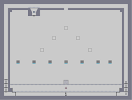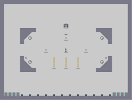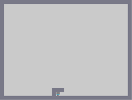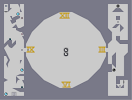Deleeecious! Don't Look Down Ornamental CEIZURE Time Travel

Pages: (0)

### wtf....

i agree with it...

### @ setthestorm

What????????????????

### OMG

CAN'T YOU SEE! THE IDEA IS THAT THE DRONES COME DOWN AS HUMANS WOKEN UP BY A BURGLAR!!!! THE KEYS IN THE BATHROOM ARE THERE TO SEEM STUFF THAT ARE IN BATHROOMS... SINK,SHOWER... ARE YOU STUPID??

http://numa.notdot.net/map/22758

### nevermind

mines rated number 4 again

by the time i posted that message...this one had moved...
no it wasnt from me...

### nice

very good

maybe try to make the eye more noticable?
same with the feet...
i wish people wouldn tpost the same thing three times...
especially when they are full of shit...try looking at the one thats rated number one, or even mine which is rated...i think the next below this one(granted mine isnt extremely good, but hell, people seem to like it)

anyway, great for your first, keep them coming

### i hate n art

the only decent piece i have ever seen

### i hate n art

the only decent piece i have ever seen

### i hate n art

the only decent piece i have ever seen

### 5/5

Pretty good; Must have taken FOREVER